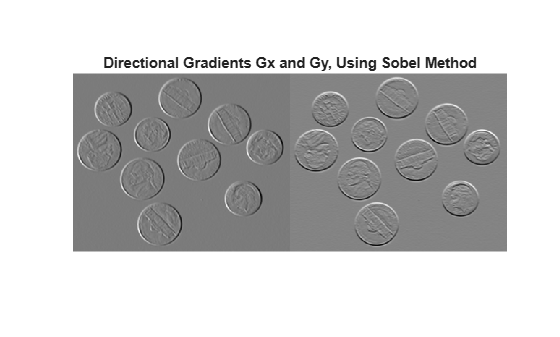## 语法

``[Gx,Gy] = imgradientxy(I)``
``[Gx,Gy] = imgradientxy(I,method)``

## 说明

``[Gx,Gy] = imgradientxy(I)` 返回灰度或二值图像 `I` 的定向梯度 `Gx` 和 `Gy`。`

``[Gx,Gy] = imgradientxy(I,method)` 使用指定的 `method` 返回定向梯度。`

## 示例

`I = imread('coins.png');`

`[Gx, Gy] = imgradientxy(I,'prewitt');`

```figure imshowpair(Gx, Gy, 'montage'); title('Directional Gradients: x-direction, Gx (left), y-direction, Gy (right), using Prewitt method')````I = imread('coins.png');`

`[Gx,Gy] = imgradientxy(I);`

```imshowpair(Gx,Gy,'montage') title('Directional Gradients Gx and Gy, Using Sobel Method')````[Gmag,Gdir] = imgradient(Gx,Gy);`

```imshowpair(Gmag,Gdir,'montage') title('Gradient Magnitude (Left) and Gradient Direction (Right)')```## 输入参数

`'sobel'`

Sobel 梯度算子。像素的梯度是 3×3 邻域内像素的加权和。在垂直 (y) 方向，权重为：

```[ 1 2 1 0 0 0 -1 -2 -1 ]```

`'prewitt'`

Prewitt 梯度算子。像素的梯度是 3×3 邻域内像素的加权和。在垂直 (y) 方向，权重为：

```[ 1 1 1 0 0 0 -1 -1 -1 ]```

`'central'`

`'intermediate'`

## 提示

• 当在图像边界应用梯度算子时，图像边界之外的值假定为等于最接近的图像边界值。

## 算法

`imgradientxy` 不会对梯度输出进行归一化。如果梯度输出图像的范围必须与输入图像的范围匹配，请考虑对梯度图像进行归一化，具体取决于所使用的 `method` 参数。例如，对于 Sobel 核，归一化因子是 1/8，对于 Prewitt 是 1/6。